# What are Negative Stiffness Vibration Isolators?

A revolutionary concept in low-frequency vibration isolation is utilized in Minus K® vibration isolators.Vertical-motion isolation is supplied by a negative-stiffness mechanism (NSM) combined with a stiff spring that supports a weight load. The net vertical stiffness is made extremely low without influencing the static load-supporting capability of the spring.

Without influencing the static load-supporting capability of the spring, the net vertical stiffness is made extremely low. Horizontal-motion isolation is supplied by beam-columns connected in series with the vertical-motion isolator.

The horizontal stiffness of the beam-columns is decreased via the "beam-column" effect (a beam-column behaves as a spring combined with an NSM). The result is a compact passive isolator which is capable of very high internal structural frequencies and extremely low horizontal and vertical natural frequencies.

## Negative Stiffness Function

Minus K® isolators generally utilize three isolators stacked in series: a tilt-motion isolator on top of a horizontal-motion isolator on top of a vertical-motion isolator. Figure 1 shows a vertical-motion isolator.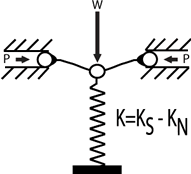Figure 1

It utilizes a conventional spring connected to a NSM which is made up of two flexures which are connected at their inner ends to the spring and supported at their outer ends, and loaded in compression by forces P. As shown in Figure 1, the spring is compressed by weight W to the operating position of the isolator.

The stiffness of the isolator is K=KS-KN where KN is the magnitude of a negative stiffness which is a function of the design of the flexures and the load P, and KS is the spring stiffness. While the spring supports the weight W, the isolator stiffness can be made to approach zero.

Figure 2 shows a horizontal-motion isolation system which is made up of two beam-column isolators. Each isolator acts like two fixed-free beam columns loaded axially by a weight load W. The beam-columns have horizontal stiffness KS without the weight load. With the weight load, the lateral bending stiffness is decreased via the "beam-column" effect.

This behavior is the same as an NSM combined with a horizontal spring so that the horizontal stiffness is K=KS-KN, and KN is the magnitude of the beam-column effect. By loading the beam-columns to approach their critical buckling load, horizontal stiffness can be made to approach zero.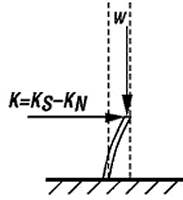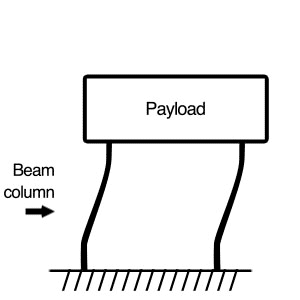Figure 2

A schematic of a negative-stiffness vibration isolator, which incorporates the isolators of Figures 1 and 2, can be observed in Figure 3. A tilt pad acts as the tilt-motion isolator and a vertical stiffness adjustment screw is utilized to modify the compression force on the negative-stiffness flexures which alters the vertical stiffness.

To adjust for varying weight loads, a vertical load adjustment screw is utilized to raise or lower the base of the support spring to hold the flexures in their unbent, straight, operating position.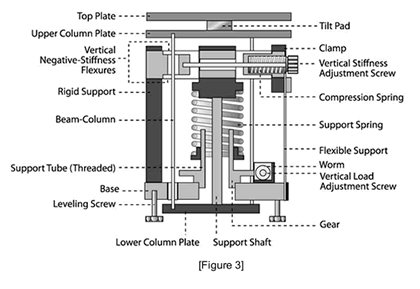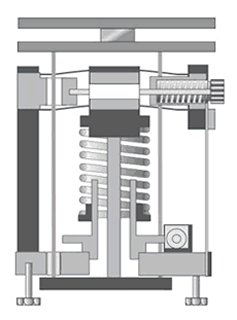Figure 3

A schematic of a typical cantilevered horizontal-motion flexure that permits a low isolator height, and low horizontal natural frequencies, can be seen in Figure 4; bearing a weight load W on its end, the flexure has x and y displacement capability.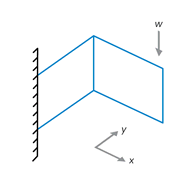Figure 4

This information has been sourced, reviewed and adapted from materials provided by Minus K Technology.## Citations

• APA

Minus K Technology. (2019, October 29). What are Negative Stiffness Vibration Isolators?. AZoM. Retrieved on August 09, 2020 from https://www.azom.com/article.aspx?ArticleID=15733.

• MLA

Minus K Technology. "What are Negative Stiffness Vibration Isolators?". AZoM. 09 August 2020. <https://www.azom.com/article.aspx?ArticleID=15733>.

• Chicago

Minus K Technology. "What are Negative Stiffness Vibration Isolators?". AZoM. https://www.azom.com/article.aspx?ArticleID=15733. (accessed August 09, 2020).

• Harvard

Minus K Technology. 2019. What are Negative Stiffness Vibration Isolators?. AZoM, viewed 09 August 2020, https://www.azom.com/article.aspx?ArticleID=15733.

## Tell Us What You Think